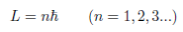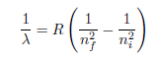# 2015PUPC普林斯顿大学物理竞赛Online真题与答案免费下载

### Online线上竞赛部分### Online Part 完整版真题免费下载

Online线上竞赛部分此卷共3大题

Atomic Physics is one of the most studied topics in Physics. Beginning in the late 1800s, it has advanced our understanding of the universe. From the discovery of the atom as the fundamental unit of matter to the nuclear reactor, atomic physics has been growing exponentially for the last century. One of the most impor­tant discoveries to date is the use of laser cooling to slow down atoms. The current record of atomic cooling is on the order of pico-Kelvins, or 12 orders of magnitude less than a Kelvin. The method of laser cooling has enabled us to observe many new phenomena. Research in Bose-Einstein condensation, spectroscopy, and even quantum computers requires laser cooling.

Through this competition, students are introduced to various methods in atomic physics centered around the concept of laser cooling. Students will derive concepts from both the semi-classical and quantum me­chanical views of atomic theory. Most of the problems require the students to re-derive the given equations, for these problems students are graded from the work shown in derivation steps. For some problems that require visualization, students are expected to show clear scales on their graph. Problems that require the use of a computational device will always be clearly indicated.

Each section of the background material is followed by several conceptual and/or applied questions that you are expected to answer in any order you choose (while the material is structured in a way that would lead you through the assignment sequence logically). All of the problems here should be able to be solved using only mechanics and electromagnetism, while new concepts will always explained.

These problems cover Nobel prizes beginning with Zeeman in 1902 for the Zeeman Split, continuing with Bohr in 1922 for the Bohr atomic model, Rabi for Rabi oscillations in 1944, Bloch for the optical Bloch equation (and a great last name) in 1952, and Chu, Cohen-Tannoudji, and Phillips in 1997 for laser cooling.

These are very advanced topics, some of which are only taught in advanced undergraduate courses. We expect very few teams to ﬁnish the entire packet. We expect that when students ﬁnd concepts that are unfamiliar, they will be resourceful and research topics using allowed resources that do not violate the collaboration policy. Completing any one of the parts is a major accomplishment for a team, let alone ﬁnishing all three parts.

Good luck!

1. #### Introduction

1.1 Bohr Atom

In 1913 Bohr postulated that the atom can be viewed as a tiny positive charged nucleus, with the electron circulating around it, similar to the earth rotating around the sun. We need to remember that circulating charged material will radiate away its energy; thus, the electron will fall into a lower energy orbit. In his postulate, Bohr suggest that the orbit follows a certain quantum mechanical pattern that we will discuss here. In this section, we will determine what we mean when we say “excited” or “ground” state of an atom.

Consider an atom of hydrogen, consisting of a proton of mass mp and an electron of mass me, in a vacuum and isolated from other atoms. In this problem, we will derive the spectrum of hydrogen in the semi-classical limit.

1.Suppose the electron orbits the proton in a circular orbit of radius r. Write down the forces that the electron experiences.

2.Write down the equations of motion for the system. Do not assume that mp > me.

3.In this classical derivation we can see that r can assume the value of any real number; thus, the spectrum of the hydrogen atom should be continuous. However in 1871 Angstrom measured 4 discrete spectra of Hydrogen:Figure 1: Table of hydrogen spectrum observed by Angstrom

Bohr postulated in 1913 that the angular momentum of the electron is discrete and given by:where h is the reduced Planck constant.
From this assumption, derive the possible energy levels of the electron.
4. From these discrete energy levels, derive the possible radial separations between the proton and the electron r. Show that it will take the form ofwhere RE is a constant. Find RE in terms of fundamental constants.
5. From this derivation, we can arrive at the formula that was known before Bohr's assumption, known as the Rydberg formula. Remember that the energy of a photon with frequency f is Eph = hf. Show that the possible spectra wavelengths  can be given in the following form:for some constant R, with nf ; ni being positive integers. Find R in terms of fundamental constants.
6. From the Rydberg formula, nd the pair nf , ni that corresponds to the wavelength  λ that Angstrom observed.